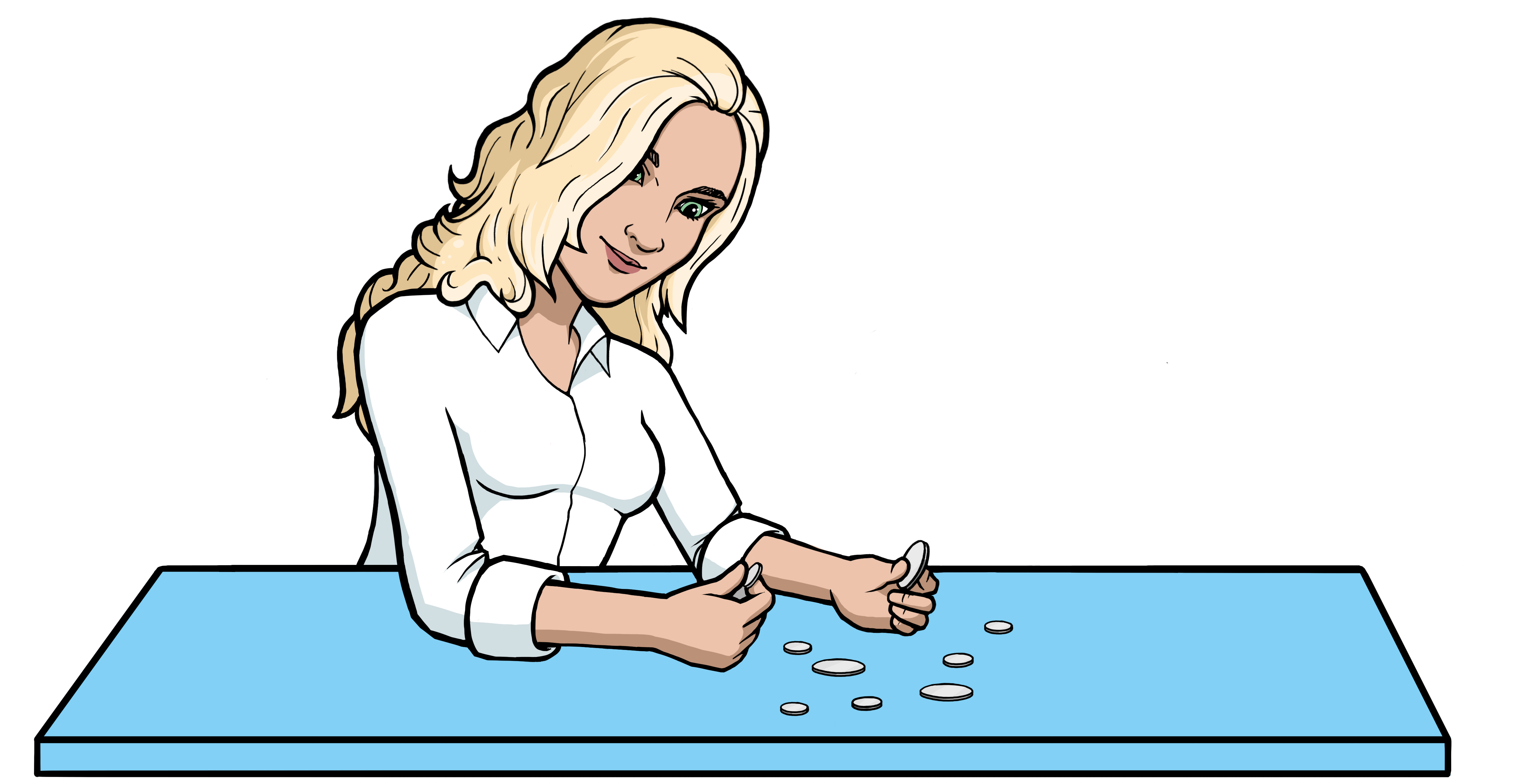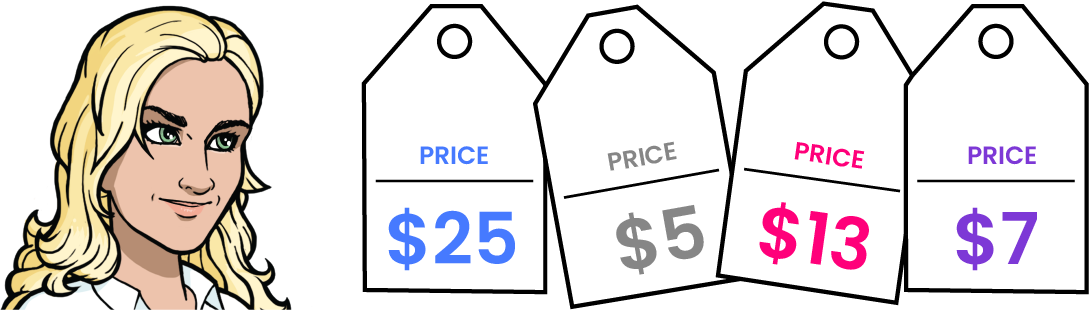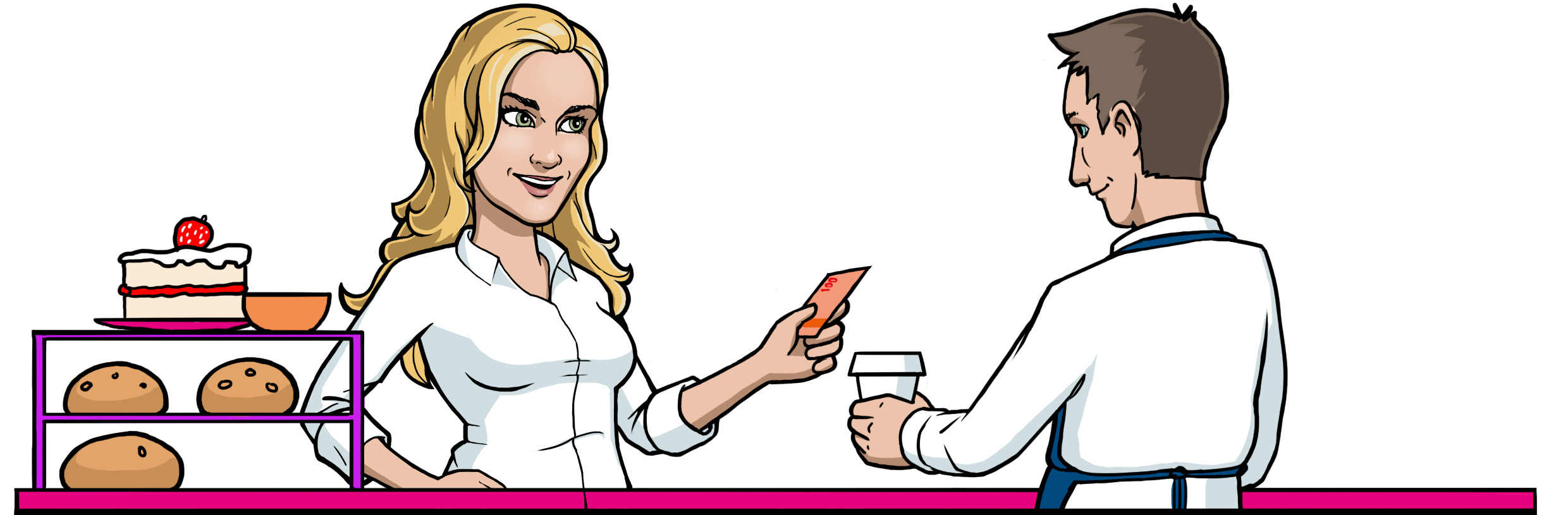Math Topics

# How Math Is Used in Shopping

Buying and selling are activities that become a bigger part of your daily life as you grow older. That’s why it’s important for you to understand how it works.

When you buy or sell something, you trade money for an item or service. You can give or receive the money as coins and bills, or it can be sent directly to a bank account.

Here are some examples of how to use coins and bills together.Example 1

An app costs $\text{}\text{}2.70\text{}$. Which coins can you use to pay for it?

$\begin{array}{llll}\hfill \text{}2.70& =2\cdot \text{}1+70\phantom{\rule{0.17em}{0ex}}\text{¢}\phantom{\rule{2em}{0ex}}& \hfill & \phantom{\rule{2em}{0ex}}\\ \hfill & =\text{}2+50\phantom{\rule{0.17em}{0ex}}\text{¢}+20\phantom{\rule{0.17em}{0ex}}\text{¢}\phantom{\rule{2em}{0ex}}& \hfill & \phantom{\rule{2em}{0ex}}\end{array}$

 $\text{}2.70=2\cdot \text{}1+70\phantom{\rule{0.17em}{0ex}}\text{¢}=\text{}2+50\phantom{\rule{0.17em}{0ex}}\text{¢}+20\phantom{\rule{0.17em}{0ex}}\text{¢}$

You can use two dollars, two quarters, and two dimes.

Example 2

An iPhone case is on sale for $\text{}\text{}9.80\text{}$. Which bills and coins might you use to buy the cover?

$\begin{array}{llll}\hfill \text{}9.80& =\text{}9+80\phantom{\rule{0.17em}{0ex}}\text{¢}\phantom{\rule{2em}{0ex}}& \hfill & \phantom{\rule{2em}{0ex}}\\ \hfill & =\text{}5+\text{}4+50\phantom{\rule{0.17em}{0ex}}\text{¢}+30\phantom{\rule{0.17em}{0ex}}\text{¢}\phantom{\rule{2em}{0ex}}& \hfill & \phantom{\rule{2em}{0ex}}\end{array}$

 $\text{}9.80=\text{}9+80\phantom{\rule{0.17em}{0ex}}\text{¢}=\text{}5+\text{}4+50\phantom{\rule{0.17em}{0ex}}\text{¢}+30\phantom{\rule{0.17em}{0ex}}\text{¢}$

You can use a \$$5$ bill, two \$$2$ bills, a half dollar and three dimes. You could also pay with a \$$5$ bill, four \$$1$ bills, three quarters and a nickel.

Example 3

A big bag of candy and two sodas costs $\text{}\text{}8.20\text{}$. What bills and coins might you use to pay for this?

 $\text{}8.20=\text{}5+\text{}2+\text{}1+20\phantom{\rule{0.17em}{0ex}}\text{¢}$

You can use a \$$5$ bill, a \$$2$ bill, a dollar and two dimes.Example 4

An iPhone charger costs $\text{}\text{}27.90\text{}$. Which bills and coins could you use to buy the charger?

$\begin{array}{llll}\hfill \text{}27.90& =\text{}20+\text{}7+90\phantom{\rule{0.17em}{0ex}}\text{¢}\phantom{\rule{2em}{0ex}}& \hfill & \phantom{\rule{2em}{0ex}}\\ \hfill & =\text{}20+\text{}5+\text{}2+90\phantom{\rule{0.17em}{0ex}}\text{¢}\phantom{\rule{2em}{0ex}}& \hfill & \phantom{\rule{2em}{0ex}}\\ \hfill & =\text{}20+\text{}5+\text{}2+50\phantom{\rule{0.17em}{0ex}}\text{¢}+40\phantom{\rule{0.17em}{0ex}}\text{¢}\phantom{\rule{2em}{0ex}}& \hfill & \phantom{\rule{2em}{0ex}}\end{array}$ You can use a \$$20$ bill, a \$$5$ bill, two \$$1$ bills, two quarters, and four dimes.

Example 5

A screen protector for an iPhone costs $\text{}\text{}16.70\text{}$. What bills and coins might you use to buy the protector?

$\begin{array}{llll}\hfill \text{}16.70& =\text{}16+70\phantom{\rule{0.17em}{0ex}}\text{¢}\phantom{\rule{2em}{0ex}}& \hfill & \phantom{\rule{2em}{0ex}}\\ \hfill & =\text{}10+\text{}6+70\phantom{\rule{0.17em}{0ex}}\text{¢}\phantom{\rule{2em}{0ex}}& \hfill & \phantom{\rule{2em}{0ex}}\\ \hfill & =\text{}10+\text{}5+\text{}1+70\phantom{\rule{0.17em}{0ex}}\text{¢}\phantom{\rule{2em}{0ex}}& \hfill & \phantom{\rule{2em}{0ex}}\\ \hfill & =\text{}10+\text{}5+\text{}1+50\phantom{\rule{0.17em}{0ex}}\text{¢}+20\phantom{\rule{0.17em}{0ex}}\text{¢}\phantom{\rule{2em}{0ex}}& \hfill & \phantom{\rule{2em}{0ex}}\end{array}$ You can use a \$$10$ bill, a \$$5$ bill, a dollar, two quarters, and two dimes.Example 6

A large pizza costs $\text{}\text{}29.83\text{}$, and delivery is an additional $\text{}\text{}8.90\text{}$. What bills and coins can you use to pay for both combined?

 $\text{}29.83+\text{}8.90=\text{}38.73$

$\begin{array}{llll}\hfill \text{}38.73& =\text{}38+73\phantom{\rule{0.17em}{0ex}}\text{¢}\phantom{\rule{2em}{0ex}}& \hfill & \phantom{\rule{2em}{0ex}}\\ \hfill & =\text{}20+\text{}10+\text{}8+73\phantom{\rule{0.17em}{0ex}}\text{¢}\phantom{\rule{2em}{0ex}}& \hfill & \phantom{\rule{2em}{0ex}}\\ \hfill & =\text{}20+\text{}10+\text{}5+\text{}3+73\phantom{\rule{0.17em}{0ex}}\text{¢}\phantom{\rule{2em}{0ex}}& \hfill & \phantom{\rule{2em}{0ex}}\\ \hfill \phantom{\text{}38.70}& =\text{}20+\text{}10+\text{}5+\text{}3\phantom{\rule{2em}{0ex}}& \hfill & \phantom{\rule{2em}{0ex}}\\ \hfill & \phantom{\rule{2em}{0ex}}+50\phantom{\rule{0.17em}{0ex}}\text{¢}+20\phantom{\rule{0.17em}{0ex}}\text{¢}+3\phantom{\rule{0.17em}{0ex}}\text{¢}\phantom{\rule{2em}{0ex}}& \hfill & \phantom{\rule{2em}{0ex}}\end{array}$

$\begin{array}{llll}\hfill \text{}38.73& =\text{}38+73\phantom{\rule{0.17em}{0ex}}\text{¢}\phantom{\rule{2em}{0ex}}& \hfill & \phantom{\rule{2em}{0ex}}\\ \hfill & =\text{}20+\text{}10+\text{}8+73\phantom{\rule{0.17em}{0ex}}\text{¢}\phantom{\rule{2em}{0ex}}& \hfill & \phantom{\rule{2em}{0ex}}\\ \hfill & =\text{}20+\text{}10+\text{}5+\text{}3\phantom{\rule{2em}{0ex}}& \hfill & \phantom{\rule{2em}{0ex}}\\ \hfill & \phantom{\rule{2em}{0ex}}+50\phantom{\rule{0.17em}{0ex}}\text{¢}+20\phantom{\rule{0.17em}{0ex}}\text{¢}+3\phantom{\rule{0.17em}{0ex}}\text{¢}\phantom{\rule{2em}{0ex}}& \hfill & \phantom{\rule{2em}{0ex}}\end{array}$

To pay for the pizza with delivery included, you can use a \$$20$ bill, a \$$10$ bill, a \$$5$, three \$$1$ bills, two quarters, two dimes, and three pennies.